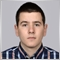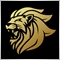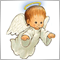# Problem with Moving Average in Custom Indicator6592

Hi guys, Im having a serious problem with calculating a MA in an indicator. In first look everything seems ok, but when attached to chart the MA goes crazy

``` int i,Counted_bars;
//--- counting from 0 to rates_total
ArraySetAsSeries(upcross,true);
ArraySetAsSeries(downcross,true);
ArraySetAsSeries(ma1,true);
ArraySetAsSeries(ma11,true);

//--- initial zero
/*if(prev_calculated<1)
{
ArrayInitialize(upcross,0);
ArrayInitialize(downcross,0);
ArrayInitialize(ma1,0);
ArrayInitialize(ma11,0);

}*/
//--------------------------------------------------------------------
Counted_bars=IndicatorCounted(); // Number of counted bars
i=Bars-Counted_bars-1;           // Index of the first uncounted
while(i>=0)
{
ma1[i]=ma(i,timeframe);
ma11[i]=ma2(i,timeframe);
if(ma(i,timeframe)<=close(i,timeframe) && Close[i]>Open[i])
{
upcross[i]=low(i,0);
}
else if(ma2(i,timeframe)>=close(i,timeframe) && Close[i]<Open[i]) downcross[i]=high(i,timeframe);
i--;
}
//--- return value of prev_calculated for next call
return(rates_total);
}
//+------------------------------------------------------------------+
//|                                                                  |
//+------------------------------------------------------------------+
double ma(int shift,ENUM_TIMEFRAMES tf)
{
double ma=iMA(Symbol(),tf,maperiod,shift,mamethod,PRICE_HIGH,shift);
return ma;
}
double ma2(int shift,ENUM_TIMEFRAMES tf)
{
double ma=iMA(Symbol(),tf,maperiod,shift,mamethod,PRICE_LOW,shift);
return ma;
}  ```Moderator
12666

That's odd it should not be drawing any arrows.6592

Marco vd Heijden:
That's odd it should not be drawing any arrows.

why not ?3010

Stanislav Ivanov

Hi guys, Im having a serious problem with calculating a MA in an indicator. In first look everything seems ok, but when attached to chart the MA goes crazy

At least you should change this:

```ma=iMA(Symbol(),tf,maperiod,shift,mamethod,PRICE_...,shift);
```

I suppose you want to use something like this:

`ma=iMA(Symbol(),tf,maperiod,0,mamethod,PRICE_...,shift);`6592

Petr Nosek:

At least you should change this:

I suppose you want to use something like this:

Worked, Thank you!